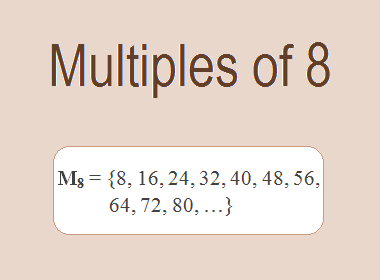# Multiples of 8The Multiples of 8 are 8, 16, 24, 32, 40, 48, 56, 64, 72, 80, … i.e. M8 = {8, 16, 24, 32, 40, 48, 56, 64, 72, 80, …}. Multiples of 8 are the series of numbers obtained by multiplying 8 with the natural numbers.

8 × 1 = 8

8 × 2 = 16

8 × 3 = 24

8 × 4 = 32

8 × 5 = 40

8 × 6 = 48

8 × 7 = 56

8 × 8 = 64

8 × 9 = 72

8 × 10 = 80

And so on.

From this series, we observe that the multiples of 8 can be derived by multiplying 8 by natural numbers in ascending order. These multiples form an arithmetic sequence with a common difference of 8.

## Properties and Patterns of Multiples of 8

1.    Evenness: One remarkable property of the multiples of 8 is that they are all even numbers. This stems from the fact that 8 itself is an even number, divisible by 2. When any integer is multiplied by 8, the resulting multiple will always be even.

2.    Repeating Digits: Upon closer examination, we uncover an intriguing pattern related to the last two digits of the multiples of 8. The last two digits follow a cyclic sequence: 08, 16, 24, 32, 40, 48, 56, 64, 72, 80. This pattern perpetually repeats itself, forming an infinite cycle.

3.    Divisibility Rule: Multiples of 8 possess a practical divisibility rule. If the last three digits of a number are divisible by 8, then the number itself is divisible by 8. This divisibility rule stems from the factors of 8: 1, 2, 4, and 8, which form a subset of the decimal system.

********************

********************

## Applications of Multiples of 8

The multiples of 8 find practical applications in various domains, including mathematics, computer science, and engineering. Here are a few notable examples:

1.    LCM: One of the applications of multiples of 8 is in finding the lowest common multiple (LCM) of two or more numbers. The LCM is the lowest multiple that two or more numbers have in common. For example, to find the LCM of 8 and 12, we need to find the multiples of both numbers and identify the lowest multiple they have in common. The multiples of 8 are 8, 16, 24, 32, 40, 48, 56, 64, 72, 80, … etc. The multiples of 12 are 12, 24, 36, 48, 60, 72, 84, 96, 108, 120 … etc. The lowest multiple that they have in common is 24. Therefore, the LCM of 8 and 12 is 24.

2.    Number Systems: Multiples of 8 play a crucial role when working with number systems beyond the decimal system. For instance, in the octal (base-8) and hexadecimal (base-16) systems, multiples of 8 hold specific values and aid in calculations and conversions.

3.    Timekeeping: In the realm of timekeeping, multiples of 8 contribute to the conversion of time units. For example, there are 8 bits in a byte, which serves as the fundamental unit for data storage and processing in computer systems.

4.    Programming and Engineering: In programming and engineering, multiples of 8 play a vital role in memory alignment and performance optimization. Data structures are often arranged in multiples of 8 bytes, known as word alignment, to enhance efficiency and facilitate faster data access.

## Conclusion:

The Multiples of 8 are the numbers obtained by multiplying 8 with natural numbers. The multiples of 8 are 8, 16, 24, 32, 40, 48, 56, 64, 72, 80, … etc. The multiples of 8 have various properties, such as evenness, repeating digits, divisibility rule, etc. The multiples of 8 have several applications in mathematics, such as finding the lowest common multiple, in number systems, timekeeping, and programming and engineering.